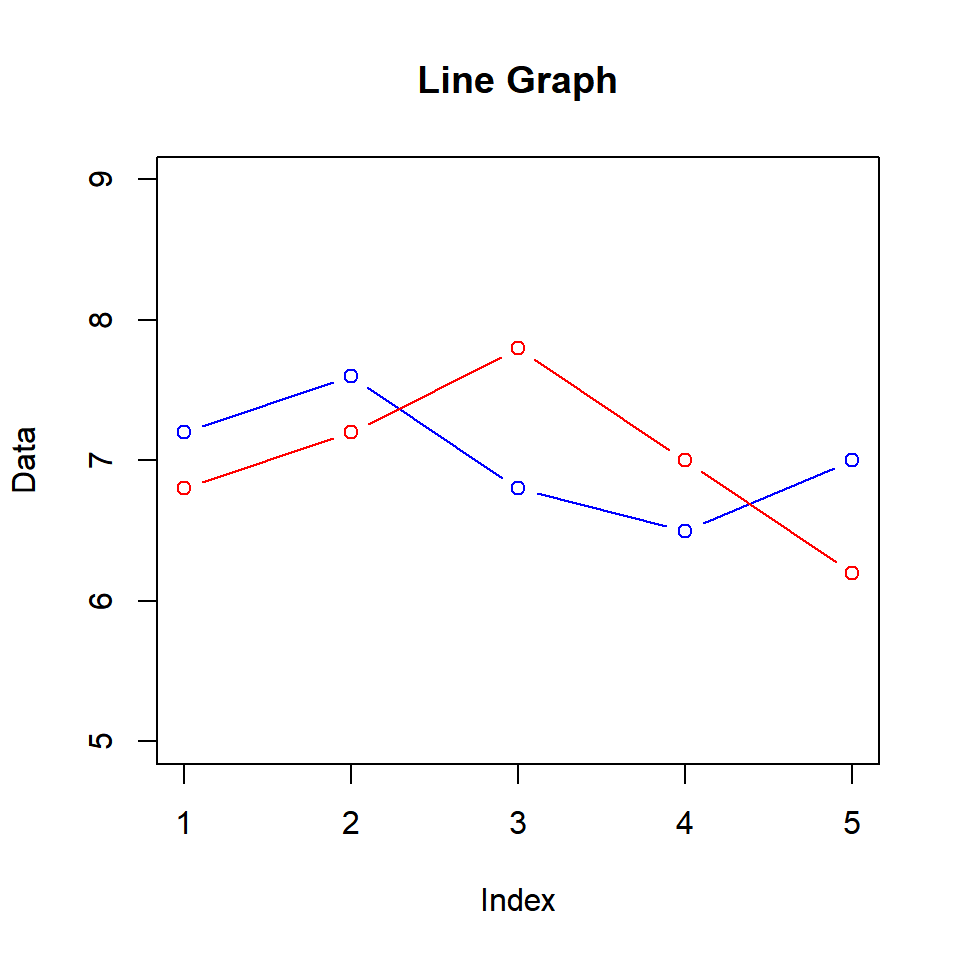# Chapter 4 Line Graphs

## 4.1 Introduction

In this chapter, we will build line graphs. To be more specific we will learn to

• create line plots
• modify line type/style
• modify line width
• add points to the lines
• modify axis range

## 4.2 Basic Plot

To build a line graph, we will learn a new argument in the `plot()` function called `type`. It allows us to specify the symbol that must be used to represent the data. Let us begin by building a simple line graph. We will use the `AirPassengers` data set in this chapter. Before we begin to build the plot, let us take a quick look at the data in order to understand what we are plotting.

``head(AirPassengers)``
``##  112 118 132 129 121 135``

In order to build a line plot, we will set the `type` argument in the `plot()` function to l (line). There are other values which `type` takes but we will explore them later.

``````data <- head(AirPassengers)
plot(data, type = 'l')``````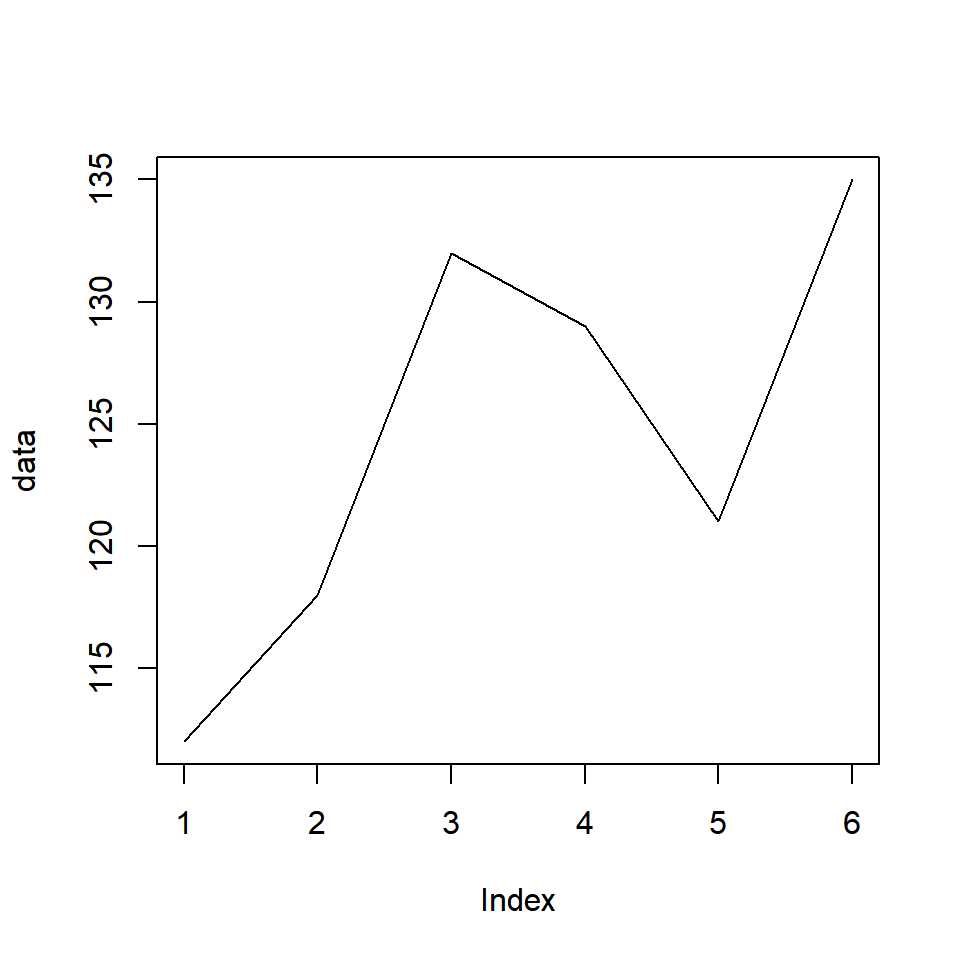If you do not like plain lines, you can represent the data using lines interspersed with points by setting the `type` argument to b (both lines and points).

``plot(data, type = 'b')``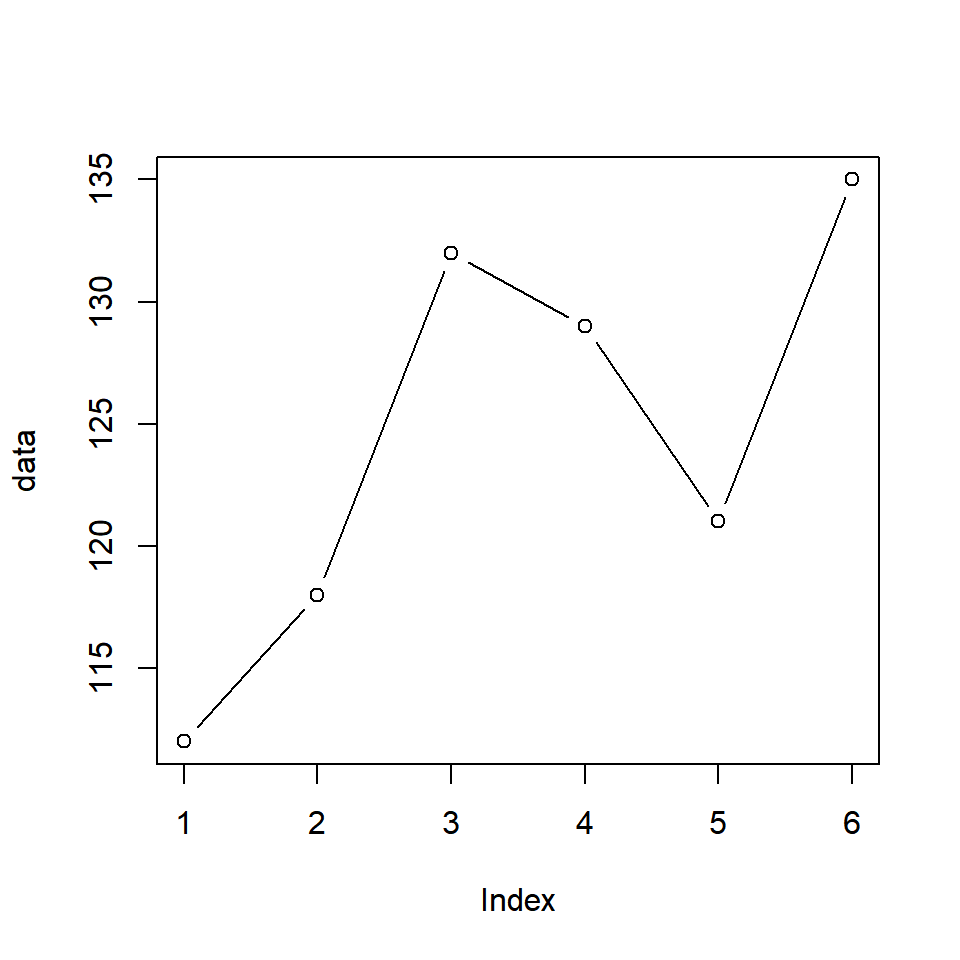Another option is to have the points and lines overplotted. It can be achieved by setting the `type` argument to o (overplotted).

``plot(data, type = 'o')``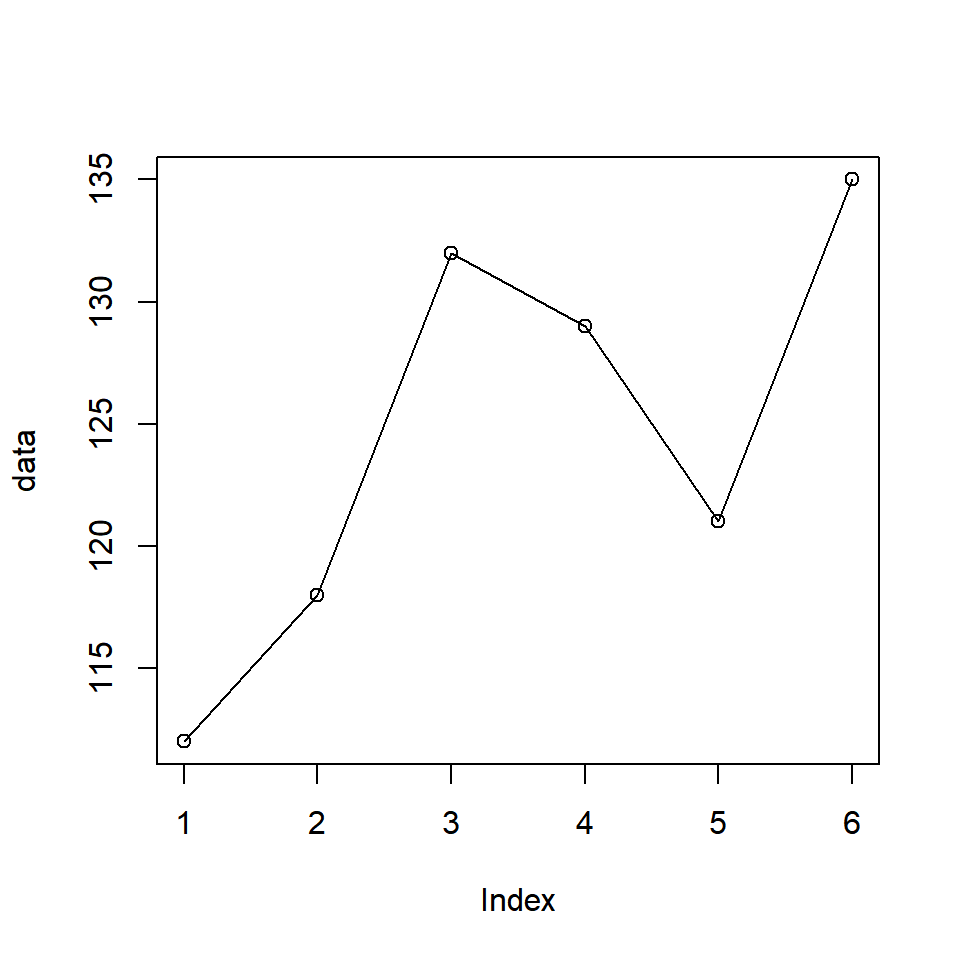You can also create lines without points but with breaks instead by setting the `type` argument to c.

``plot(data, type = 'c')``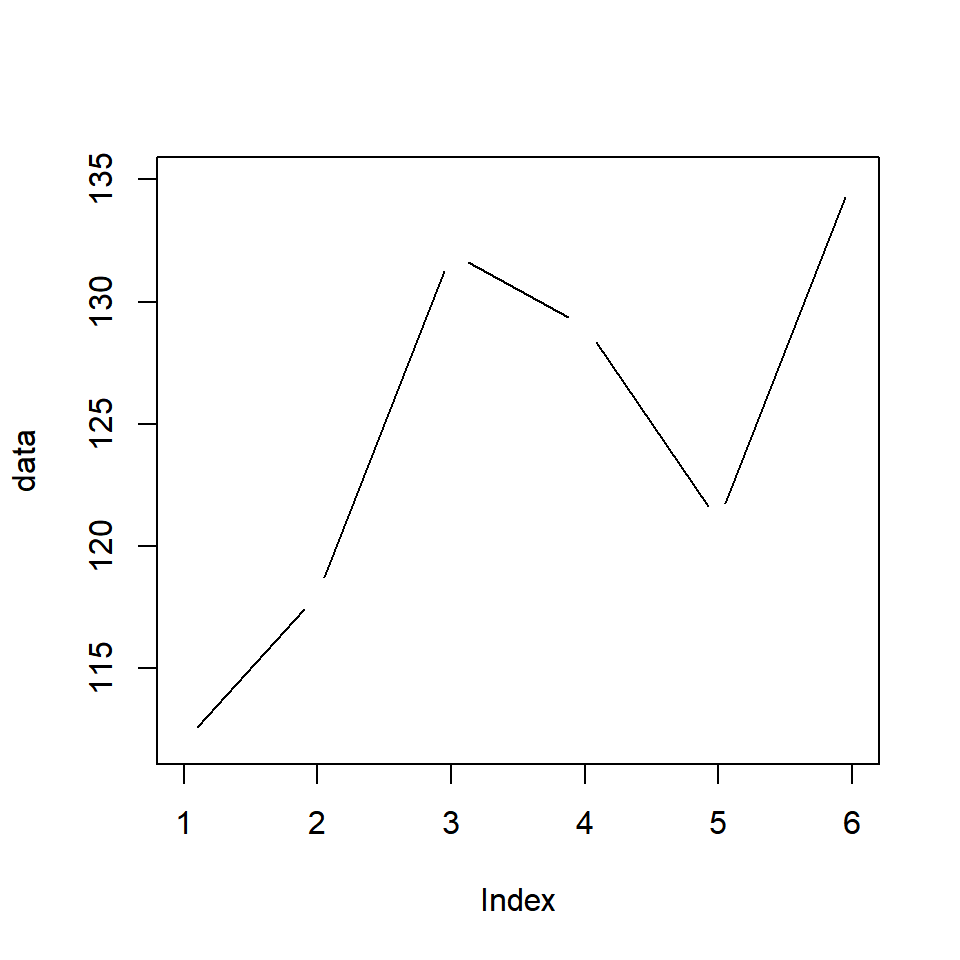## 4.3 Color

So now we know how to build a simple line graph. Let us now make this plot more elegant by modifying its appearance. Let us begin by adding some color to the line using the `col` argument in the `plot()` function.

``plot(data, type = 'l', col = 'blue')``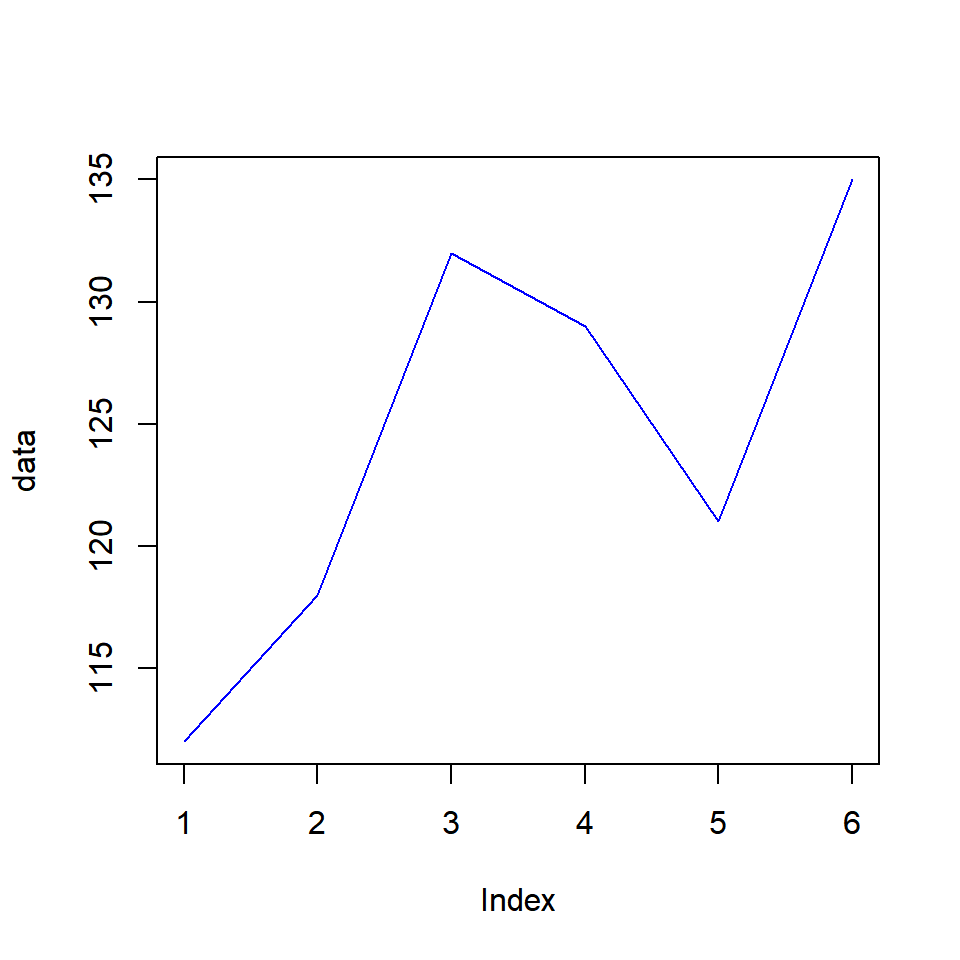If you have points along with the line, they will have the same color as well.

``plot(data, type = 'b', col = 'blue')``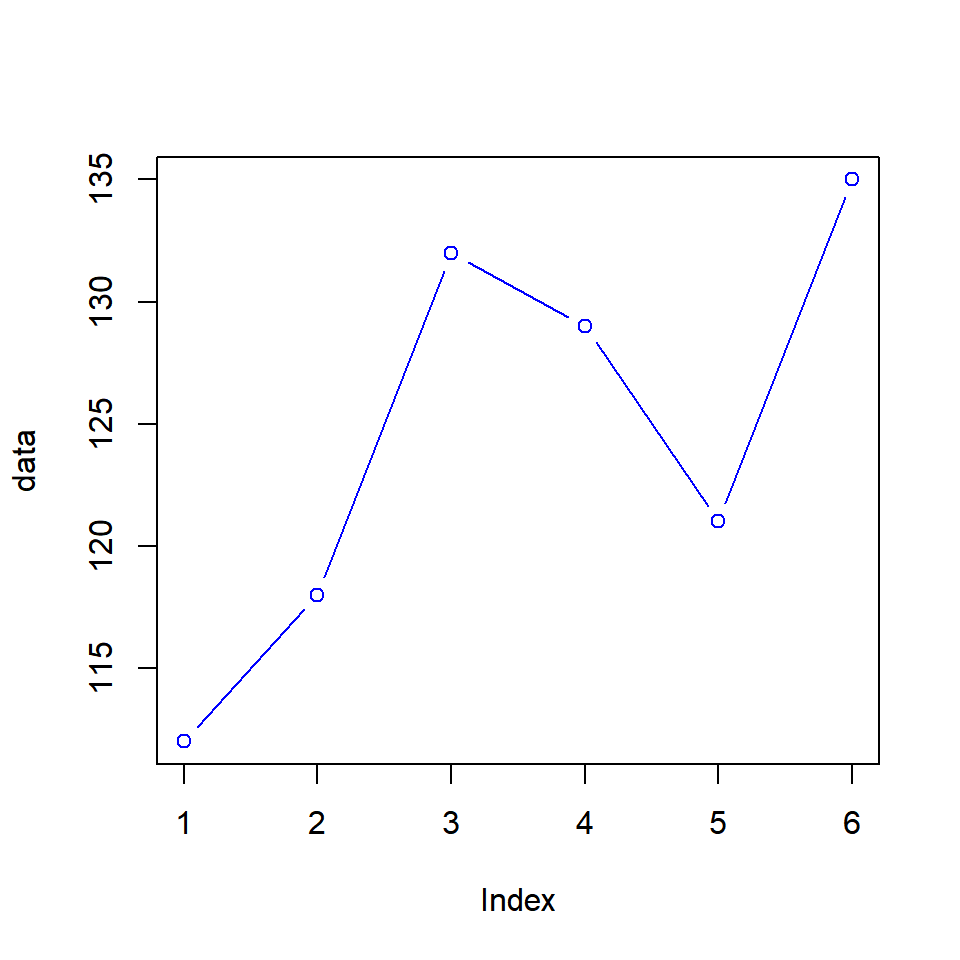## 4.4 Line Type

The line type can be modified using the `lty` argument. It takes values from 1 to 6 and the default value is 1. Below is an example:

• 1:solid
• 2:dashed
• 3:dotted
• 4:dotdash
• 5:longdash
• 6:twodash
``plot(data, type = 'l', lty = 3)``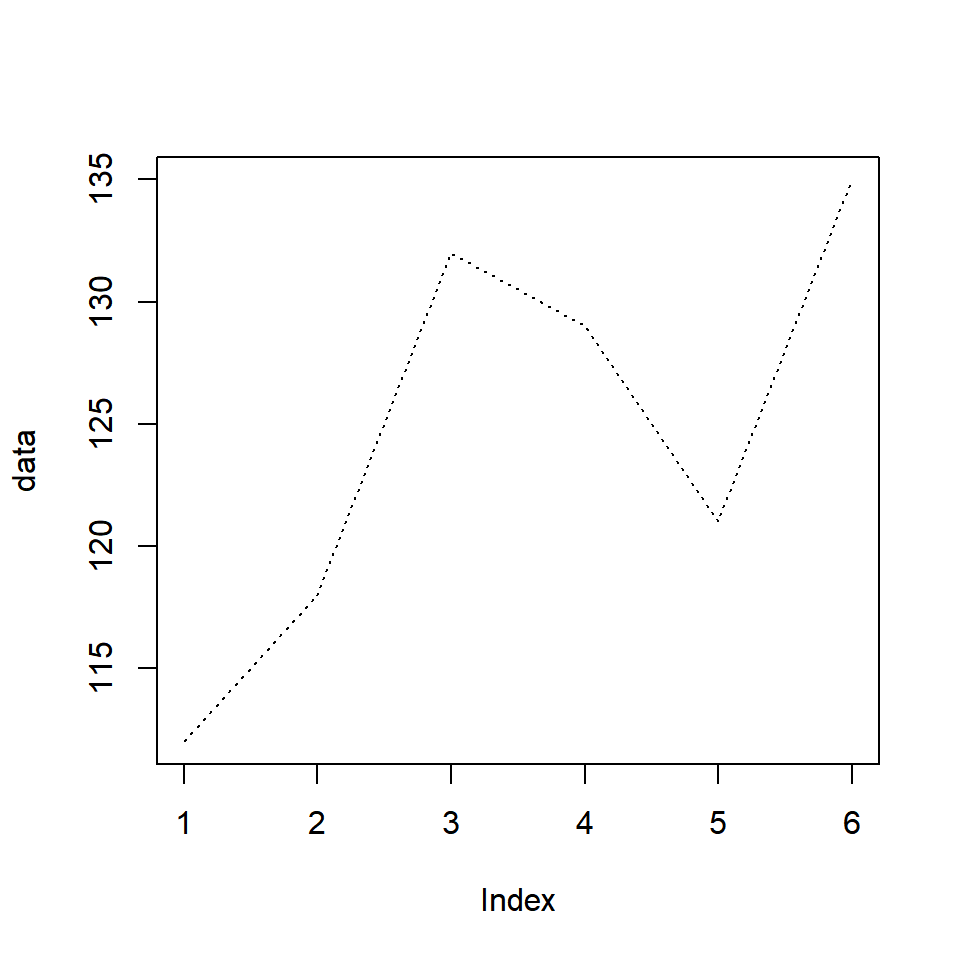Let us look at all the line types in the below example: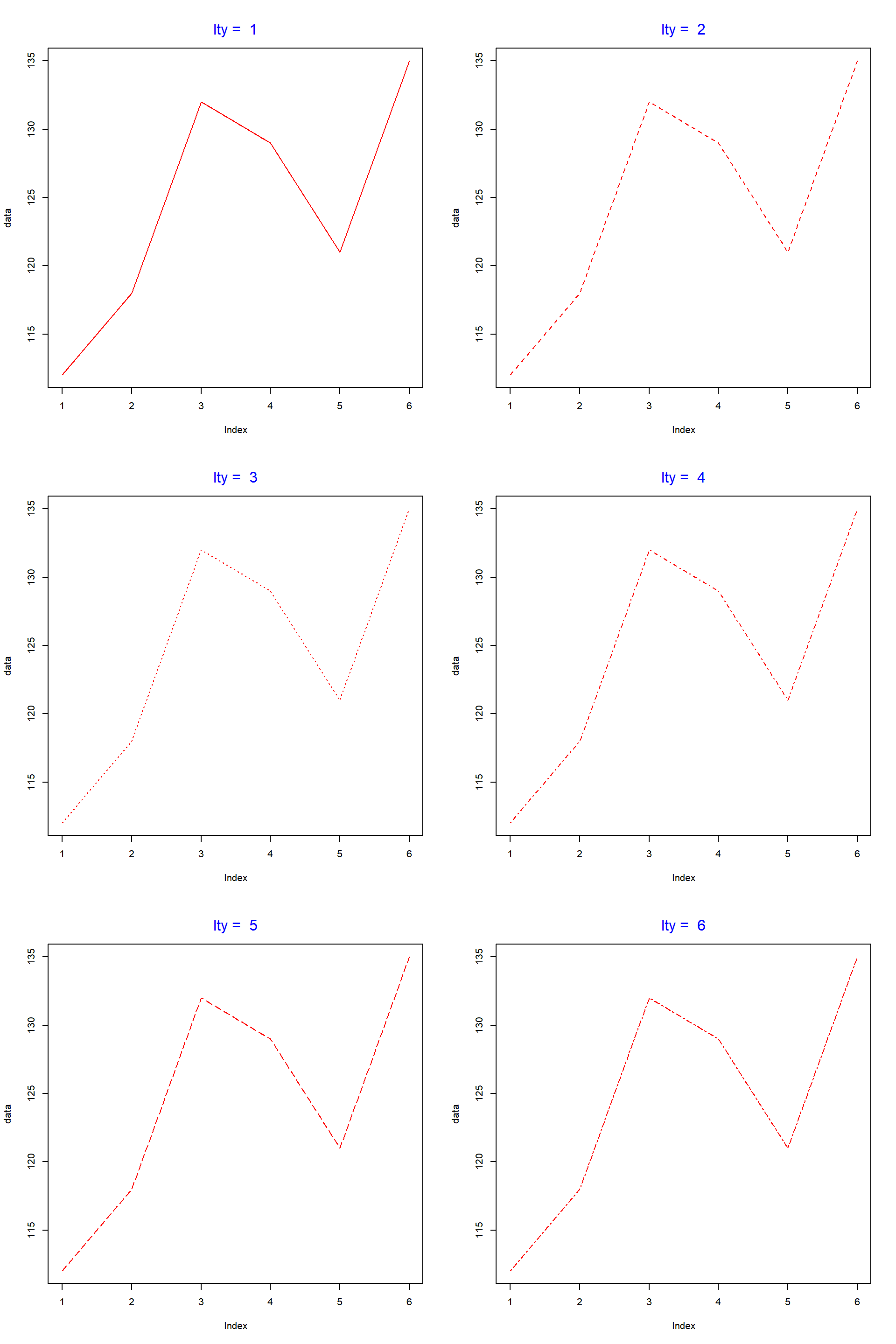Instead of specifying the numbers 1:6, you can use their description as well.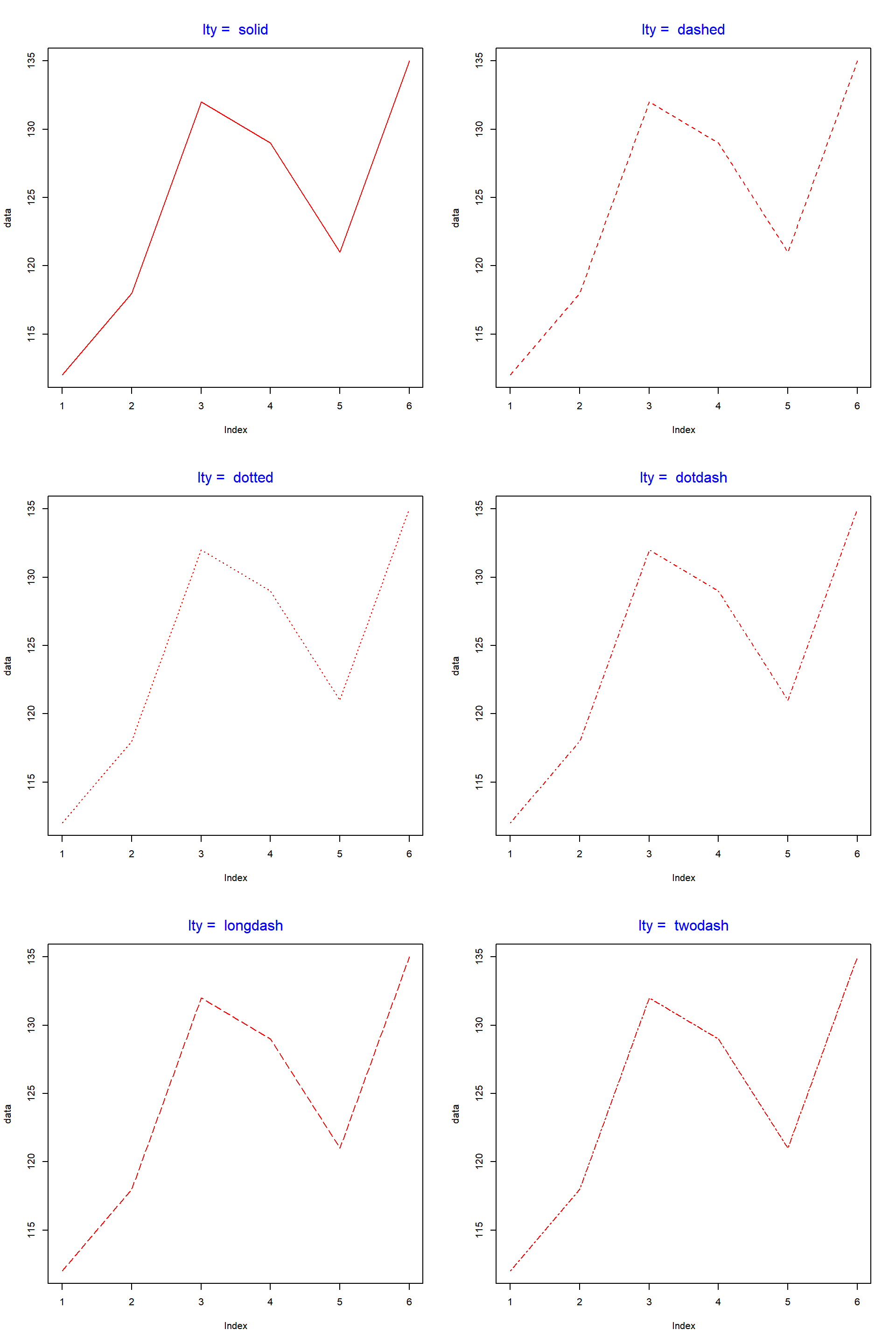## 4.5 Line Width

The width of the lines can be modified using the `lwd` argument in the `plot()` function. The default value for width is 1.

``plot(data, type = 'l', lwd = 2.5)``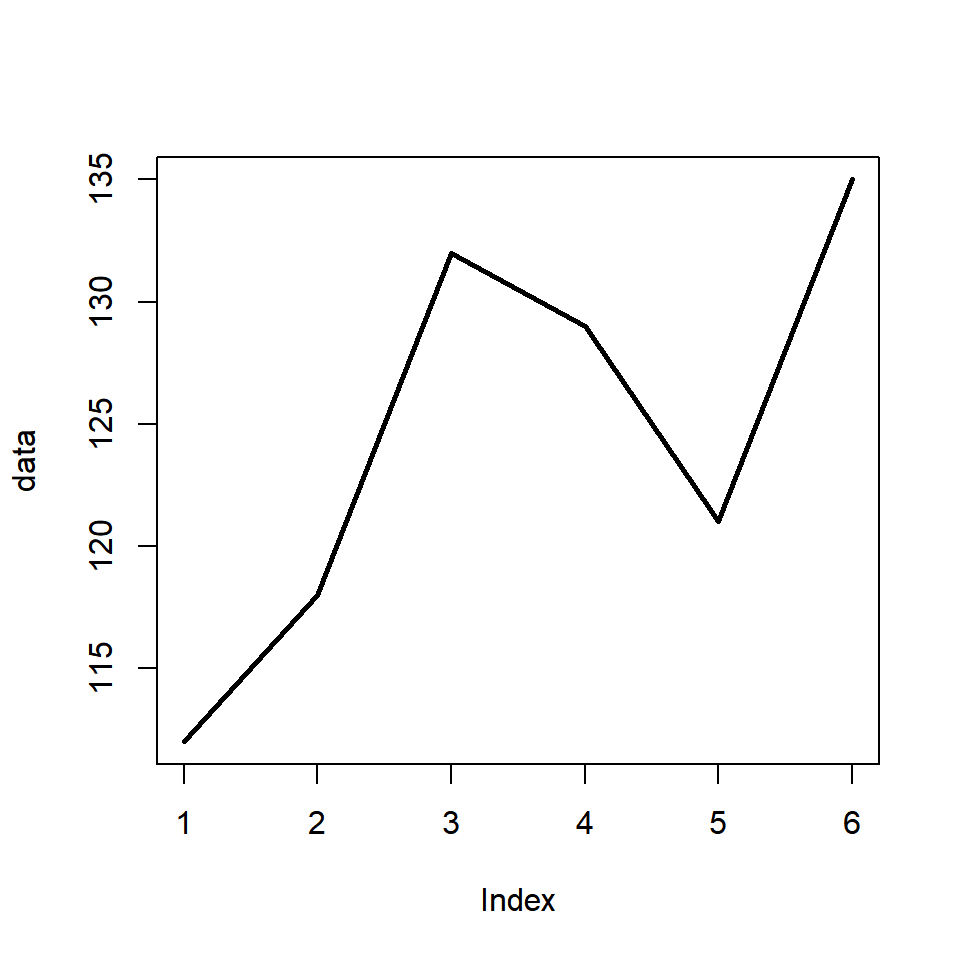In the below example, we look at the width of the lines relative to the default value.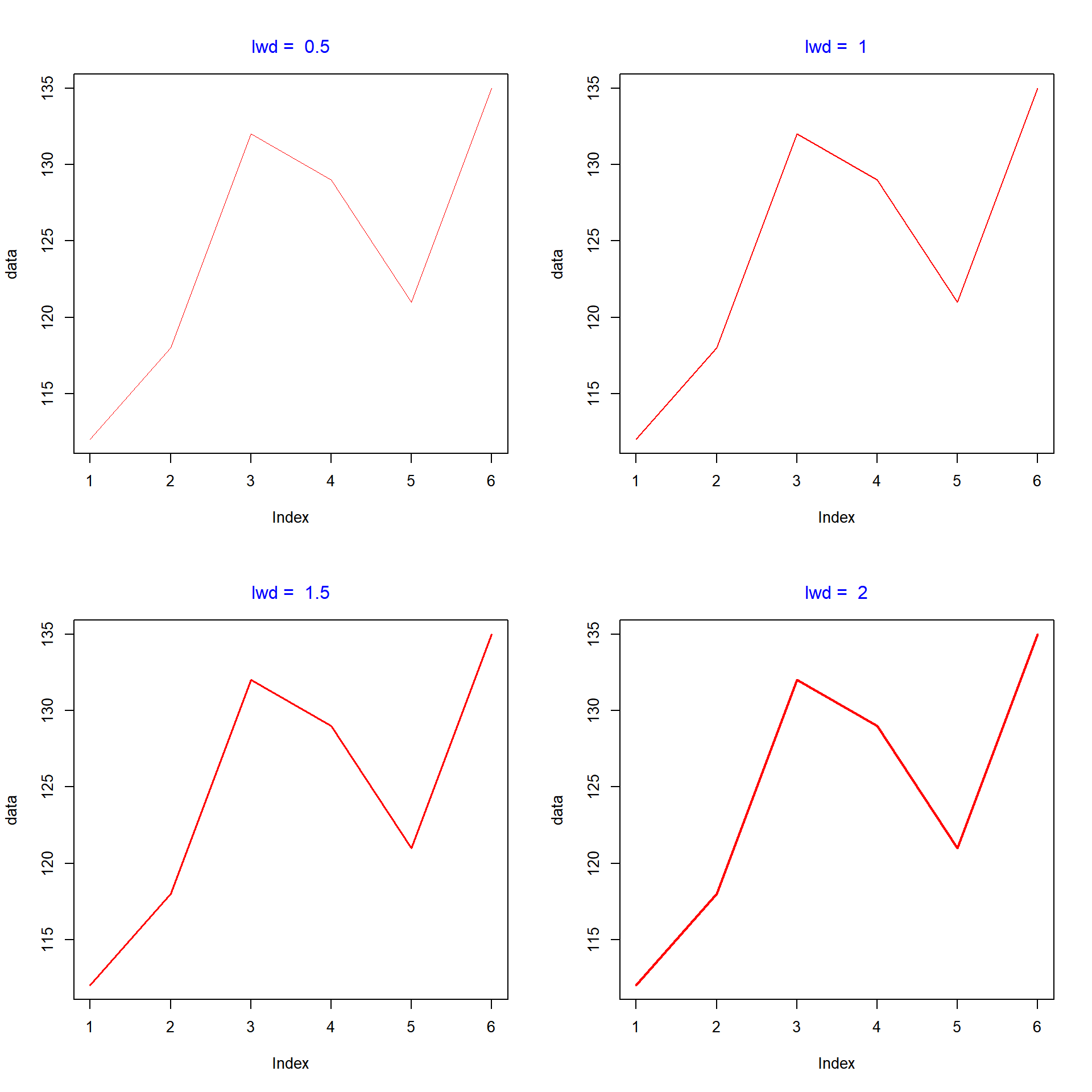## 4.6 Enhance Points

We can enhance the points in the line plot in the same way as we enhanced the points in the scatter plot in this previous chapter. Let us look at an example:

``plot(data, type = 'b', pch = 23, col = 'red', cex = 1.5)``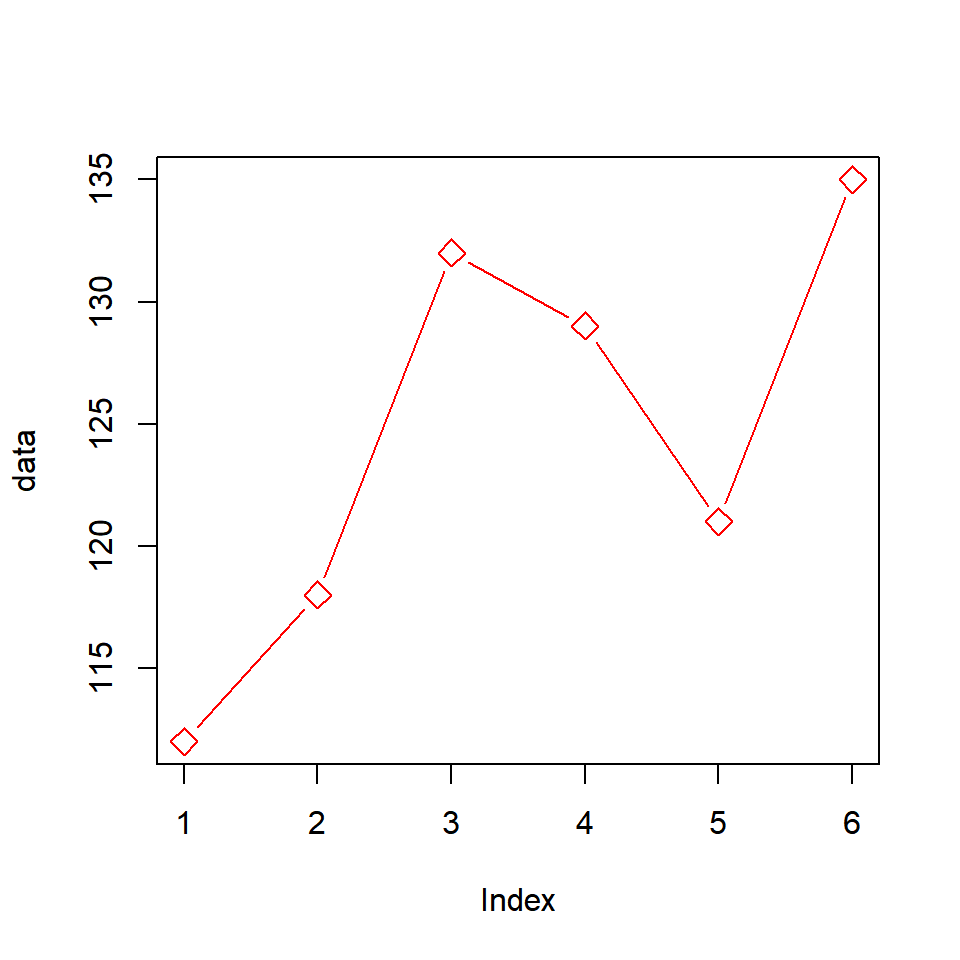We have used the `pch`, `col` and `cex` arguments to modify the shape, color and size of the points. One drawback of the above method is that the color of the line and the points will be the same. What if we want them to have different colors? The solution is as follows:

• build the line graph using the `plot()` function
• add the points to the above plot using the `points()` function

In the next example, let us first build the line plot, add points using the `points()` function and then specify separate colors to the line and the points.

``````plot(data, type = 'l', col = 'red')
points(data, pch = 23, col = 'blue', bg = 'green', cex = 1.5)``````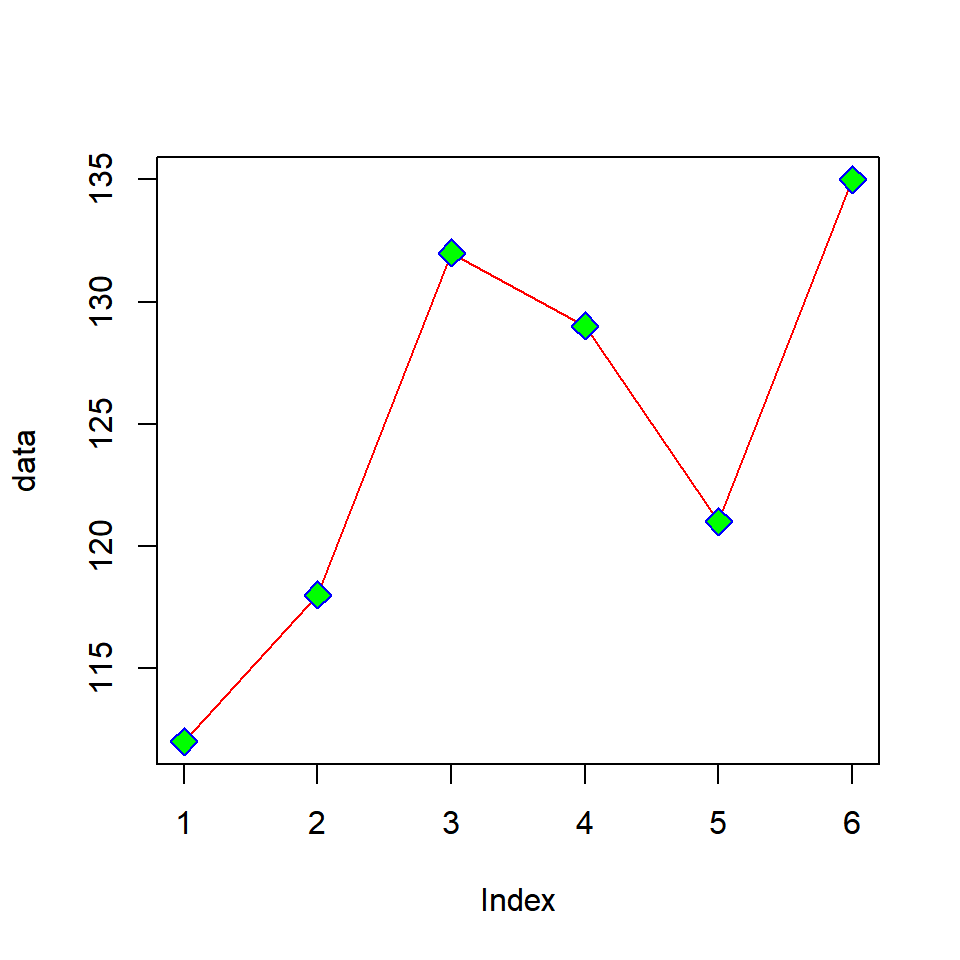If you want to compare variables, you would want to add additional lines to the line graph. In R, this can be achieved using the `lines()` function. First we create the line plot using the base variable and then we can add as many lines as we want using the `lines()` function.

Before you add additional lines, it is important to ensure that the range of both the axis are modified to accommodate the data of the additional lines. If we do not modify the axis range, some of the lines will be outside the plot.

Let us now create a line plot and add an additional line using the `lines()` function.We will use some dummy data for this example:

``````data1 <- c(7.2, 7.6, 6.8, 6.5, 7)
data2 <- c(6.8, 7.2, 7.8, 7, 6.2)
plot(data1, type = "b", col = "blue")
lines(data2, type = "b", col = "red")``````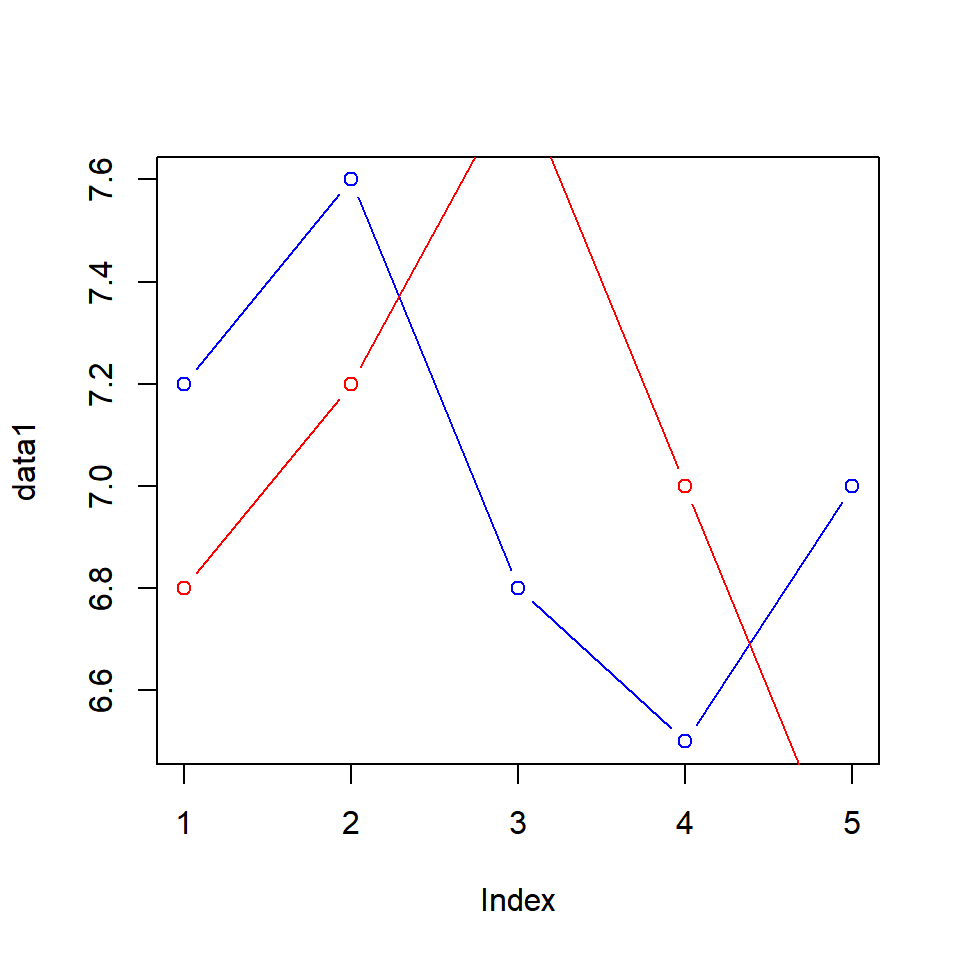As you can see the second line is outside the plot. Let us recreate the plot but this time we will modify the range of the axis to accommodate the second line (data2).

``````plot(data1, type = "b", col = "blue", ylim = c(5, 9))
lines(data2, type = "b", col = "red")``````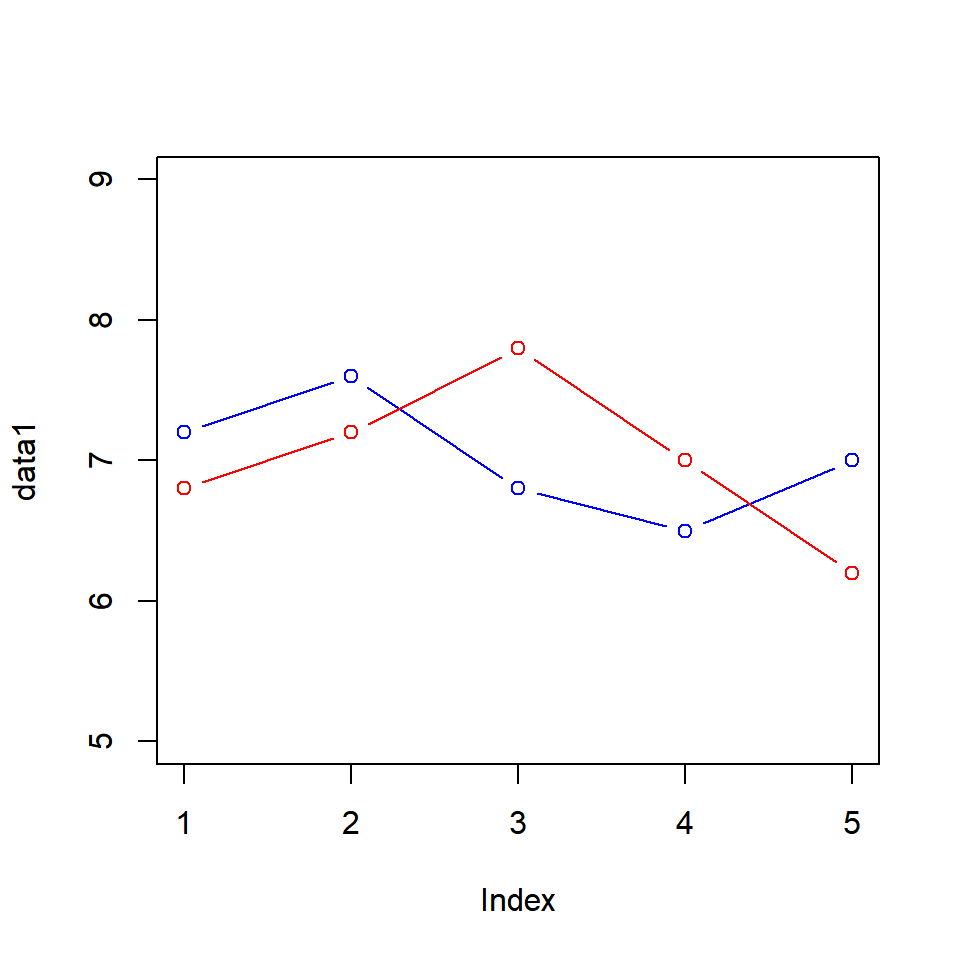## 4.8 Putting it all together…

Finally let us enhance the plot by adding a title and modifying the axis labels.

``````plot(data1, type = "b", col = "blue",
ylim = c(5, 9), main = 'Line Graph',
xlab = 'Index', ylab = 'Data')
lines(data2, type = "b", col = "red")``````